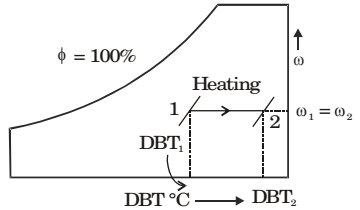## Refrigeration and Air-conditioning Miscellaneous

#### Refrigeration and Air-conditioning

1. Moist air is treated as an ideal gas mixture of water vapor and dry air (molecular weight of air = 28.84 and molecular weight of water = 18). At a location, the total pressure is 100 kPa, the temperature is 30°C and the relative humidity is 55%. Given that the saturation pressure of water at 30°C is 4246 Pa, the mass of water vapor per kg of dry air is ______ grams.

1.  w = 0.622 Pv P - Pv

 φ = Pv Ps

 ⇒ 0.55 = Pv Ps

⇒ Pv = 2235.3
 w = 0.622 × 2235.3 - 2235.3 (100 × 103)

w = 0.0142 kg/kg of dry air
w = 14.2 gm/kg of dry air

##### Correct Option: A

 w = 0.622 Pv P - Pv

 φ = Pv Ps

 ⇒ 0.55 = Pv Ps

⇒ Pv = 2235.3
 w = 0.622 × 2235.3 - 2235.3 (100 × 103)

w = 0.0142 kg/kg of dry air
w = 14.2 gm/kg of dry air

1. A stream of moist air (mass flow rate = 10.1 kg/s) with humidity ratio of 0.01 kg/kg dry air mixes with a second stream of superheated water vapour flowing at 0.1 kg/s. Assuming proper and uniform mixing with no condensation, the humidity ratio of the final stream in (kg/kg dry air) is ______.

1.  ωnew = m1ω1 + m2ω2 m1 + m2

 ⇒ 0.1 × 10.1 × + .1 × 1 = 0.02kg/kg dry air 10.1 + .1

##### Correct Option: A

 ωnew = m1ω1 + m2ω2 m1 + m2

 ⇒ 0.1 × 10.1 × + .1 × 1 = 0.02kg/kg dry air 10.1 + .1

1. The pressure, dry bulb temperature and relative humidity of air in a room are 1 bar, 30°C and 70%, respectively. If the saturated steam pressure at 30°C is 4.25 kPa, the specific humidity of the room air in kg of water vapour/kg dry air is

1.  RH = 0.7 = Pv and w = 0.622pv 4.25 100 - pv

##### Correct Option: C

 RH = 0.7 = Pv and w = 0.622pv 4.25 100 - pv

1. A room contains 35 kg of dry air and 0.5 kg of water vapor. The total pressure and temperature of air in the room are 100 kPa and 25°C respectively. Given that the saturation pressure for water at 25°C, is 3.17 kPa, the relative humidity of the air in the room is

1. ma = 35 kg, mv = 0.5 kg

 w = mv = 0.5 = 0.01428 mv 35

 also w = 0.622 Pv Pa

Pt = 100 kPa
 ∴ w = 0.622 × Pv Pt - Pv

 Now, 0.01428 = 0.622 ×PvPt - Pv

Pv = 2.238 kPa
Relative humidity
 φ = Pv = 2.238 × 100 Ps 3.17

φ = 70.6% = 71%

##### Correct Option: D

ma = 35 kg, mv = 0.5 kg

 w = mv = 0.5 = 0.01428 mv 35

 also w = 0.622 Pv Pa

Pt = 100 kPa
 ∴ w = 0.622 × Pv Pt - Pv

 Now, 0.01428 = 0.622 ×PvPt - Pv

Pv = 2.238 kPa
Relative humidity
 φ = Pv = 2.238 × 100 Ps 3.17

φ = 70.6% = 71%

1. If a mass of moist air in an airtight vessel is heated to a higher temperature, then

1. R.H. decreases##### Correct Option: D

R.H. decreases